Buy 4+ stickers and get 10% off. Buy 8+ stickers and get 15% off.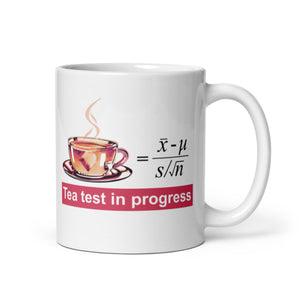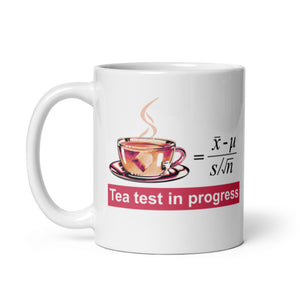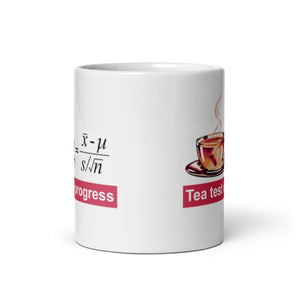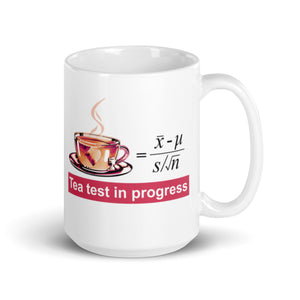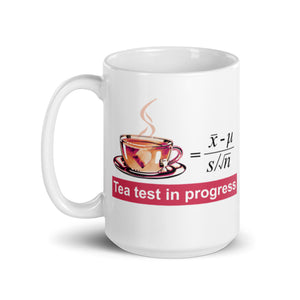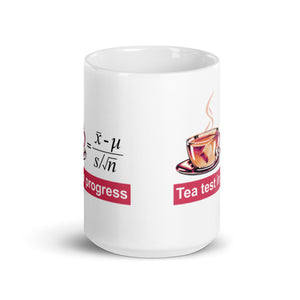Roll over image to zoom in

# Tea Test in Progress White Glossy Mug | Gift for Mathematicians and Statisticians

Regular price \$14.95 Sale

A T-test is a statistical test used to compare the means of two groups. The test statistic of this method follows a Student's t-distribution, which is used when the scale parameter (such as the population variance) is unknown. This test is commonly applied when the data would be normally distributed if the scale parameter was known. In this test, the scale parameter is estimated from the data and this results in the test statistic following a Student's t-distribution under specific conditions. The most frequent use of t-test is to check the equality of means of two groups. The T test formula is as follows.

t = ( x̄ – μ) / (s / √n)

where

•  = Observed Mean of the Sample
• μ = Theoretical Mean of the Population
• s = Standard Deviation of the Sample
• n = Sample Size

• Ceramic
• Dishwasher and microwave safe
• White and glossy finish that protects the print